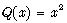6.5 Method for Using Equals

If we use equals, such as, we can define the function by substitution briefly. For example, if we descrive as follows, we can define the function Quard(x)=x·x.

definition let x be Real;
func Quard(x) equals :A1:
x·x
correctness;
end;